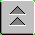####USS KEARSARGE LHD-3 SHIP'S LOADING CHARACTERISTICS PAMPHLET

1. The Secretary of the Navy has promulgated policies and procedures for use of the metric system within the Department of the Navy. In a step toward full implementation, metrics is being introduced into Ship's Loading Characteristics Pamphlets. The first phase will consist of placing metric equivalents next to or below (as space permits) the present (inch-foot) system figures. These equivalents will be placed only on cargo/vehicle/well deck stowage area diagrams and flight/HANGAR deck diagrams. Introduction of metric figures into area/volume/weight situations (both in diagrams and within charts) will be undertaken at a later date.

2. The information provided herein is intended only to acquaint personnel concerned with preparation of SLCPs with the basics of the Metric System and may require further individual research for a total understanding of the system.

3. In the Metric System there is only one series of units for length (distance), area (surface), volume (capacity) and weight (mass):

Length = Meters

Area = Square Meters In liquid measure,

the basic unit is

a liter.

Volume = Cubic Meters 1 Cubic meter = 1000 Liters

Weight/Mass = Grams/Metric Tons

RELATIVE COMPARISON

1 Meter = 39.4 inches

1 Square Meter = 1 square, 39.4 inches in length on each side

1 Cubic Meter = 1 cube, 1 square meter on each side

1 Gram = One-thousandth of a kilogram

1 Kilogram = Approximately 2.2 pounds

1 Metric Ton = Approximately 2,200 pounds

1 Liter = 1.06 quarts

4. The following symbols are utilized in the Metric System

Meter: m Metric Ton: t

Gram: g Centimeter: cm

Kilogram: kg Square Meter: m2

Liter: L (Only capital letter) Cubic Meter: m3

NOTE: No period is used after a symbol unless it appears at the end of a sentence.

5. The following rules apply when displaying numbers in the Metric System:

a. No commas are utilized (used as a decimal in some countries:

17,678 becomes 17 678

b. Numbers are shown in groupings of three when five or more are reflected (four numbers will be displayed without a space):

1,365,810 becomes 1 365 810

7,680 becomes 7 680

125.342 becomes 125.342 (no change)

.75 becomes 0.75

When the quantity being shown is less

than one, a zero must be displayed to

the left of the decimal point.

c. When numbers appear in column form, they will always be displayed in groups of three or less:

.68 becomes 0.68

8,762 becomes 8 762

+ 19,869 becomes + 19 869

CONVERSIONS

IF YOU

MULTIPLY

IF YOU HAVE YOU CAN FIND BY EXAMPLE

Inches Centimeters 2.54 6" x 2.54 = 15.24 cm

Feet Centimeters 30. 5' x 30 = 150 cm

Feet Meters 0.3 5' x 0.3 = 1.5 m

Pounds Kilograms 0.45 10lbs x 0.45 = 4.5 kg

Short Ton Metric Ton 0.9 5 s/t x 0.9 = 4.5 t

(2000 lb) (2200 lb)

Gallons Liters 3.8 5 gal x 3.8 = 19 L

Cubic Feet Cubic Meters 0.028 15 cuft x 0.028 = 0.42 m3

Square Feet Square Meters 0.0929 15 sqft x 0.0929 = 1.3935 m2

Centimeters Inches 0.4 35 cm x 0.4 = 14"

Meters Feet 3.3 12 m x 3.3 = 39.6'

Kilograms Pounds 2.2 8 kg x 2.2 = 17.6 lbs

Metric Ton Short Ton 1.1 15 t x 1.1 = 16.5 S/T

Liters Gallons 0.26 25 L x 0.26 = 6.5 gal

Cubic Meter Cubic Feet 35.3 12 m3 x 35.3 = 423.6 cuft

Square Meter Square Feet 10.8 12 m2 x 10.8 = 129.6 sqft

QUICK CHECK

Inches = Meter Feet (x .3) Meters

1 0.024 1 = 0.3

2 0.051 2 = 0.6

3 0.075 3 = 0.9

4 0.099 4 = 1.2

5 0.126 5 = 1.5

6 0.15 6 = 1.8

7 0.174 7 = 2.1

8 0.201 8 = 2.4

9 0.225 9 = 2.7

10 0.249 10 = 3.0

11 0.276 20 = 6.0

12 0.3 30 = 9.0

40 = 12.0

50 = 15.0

QUICK - CHECK IN ACTION 60 = 18.0

70 = 21.0

85 feet and 8 inches = m 80 = 24.0

90 = 27.0

80 ft = 24.0m 100 = 30.0

200 = 60.0

5 ft = 1.5m 300 = 90.0

400 = 120.0

8 in = 0.201 500 = 150.0

600 = 180.0

25.701 m 700 = 210.0

800 = 240.0

900 = 270.0

1000 = 300.0